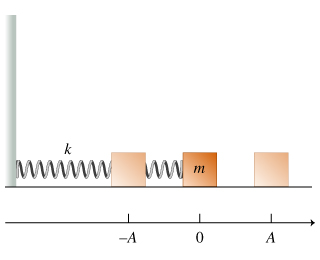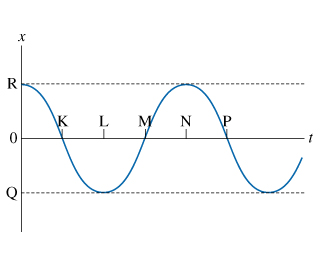# Problem: Introduction to Oscillations0 Advanced issue found▲ Figure 10 Advanced issue found▲ Figure 2The conditions that lead to simple harmonic motion are as follows:There must be a position of stable equilibrium.There must be a restoring force acting on the oscillating object. The direction of this force must always point toward the equilibrium, and its magnitude must be directly proportional to the magnitude of the object's displacement from its equilibrium position. Mathematically, the restoring force F⃗  is given by F⃗ = −kx⃗, where x⃗  is the displacement from equilibrium and k is a constant that depends on the properties of the oscillating system.The resistive forces in the system must be reasonably small.Consider a block of mass m attached to a spring with force constant k, as shown in the figure (Figure 1). The spring can be either stretched or compressed. The block slides on a frictionless horizontal surface, as shown. When the spring is relaxed, the block is located at x=0. If the block is pulled to the right a distance A and then released, A will be the amplitude of the resulting oscillations.Assume that the mechanical energy of the block-spring system remains unchanged in the subsequent motion of the block.After the block is released from x = A, it willA. remain at rest.B. move to the left until it reaches equilibrium and stop there.C. move to the left until it reaches x = −A and stop there.D. move to the left until it reaches x=−A and then begin to move to the right.

###### FREE Expert Solution

In simple harmonic motion, an object oscillates between A to -A.

90% (32 ratings)###### Problem Details

Introduction to Oscillations

0
Advanced issue foundFigure 1

0
Advanced issue foundFigure 2

The conditions that lead to simple harmonic motion are as follows:

There must be a position of stable equilibrium.

There must be a restoring force acting on the oscillating object. The direction of this force must always point toward the equilibrium, and its magnitude must be directly proportional to the magnitude of the object's displacement from its equilibrium position. Mathematically, the restoring force F⃗  is given by F⃗ = −kx⃗, where x⃗  is the displacement from equilibrium and k is a constant that depends on the properties of the oscillating system.

The resistive forces in the system must be reasonably small.

Consider a block of mass m attached to a spring with force constant k, as shown in the figure (Figure 1). The spring can be either stretched or compressed. The block slides on a frictionless horizontal surface, as shown. When the spring is relaxed, the block is located at x=0. If the block is pulled to the right a distance A and then released, A will be the amplitude of the resulting oscillations.

Assume that the mechanical energy of the block-spring system remains unchanged in the subsequent motion of the block.

After the block is released from = A, it will

A. remain at rest.
B. move to the left until it reaches equilibrium and stop there.
C. move to the left until it reaches = −A and stop there.
D. move to the left until it reaches x=−A and then begin to move to the right.

Frequently Asked Questions

What scientific concept do you need to know in order to solve this problem?

Our tutors have indicated that to solve this problem you will need to apply the Intro to Simple Harmonic Motion (Horizontal Springs) concept. You can view video lessons to learn Intro to Simple Harmonic Motion (Horizontal Springs). Or if you need more Intro to Simple Harmonic Motion (Horizontal Springs) practice, you can also practice Intro to Simple Harmonic Motion (Horizontal Springs) practice problems.